# Train Voice Activity Detection in Noise Model Using Deep Learning

This example shows how to detect regions of speech in a low signal-to-noise environment using deep learning. You train a bidirectional long short-term memory (BiLSTM) network from scratch to perform voice activity detection (VAD) and compare that network to a pretrained deep learning-based VAD. To explore the model trained from scratch in this example, see Voice Activity Detection in Noise Using Deep Learning. To use an off-the-shelf deep learning-based VAD, see `detectspeechnn`.

### Introduction

Voice activity detection is an essential component of many audio systems, such as automatic speech recognition, speaker recognition, and audio conferencing. Voice activity detection can be especially challenging in low signal-to-noise (SNR) situations, where speech is obstructed by noise.

For reproducibility, set the random seed to default.

`rng default`

In high SNR scenarios, traditional speech detection algorithms perform adequately. Read in an audio file that consists of words spoken with pauses between and listen to it.

```fs = 16e3; [speech,fileFs] = audioread("MaleVolumeUp-16-mono-6secs.ogg"); sound(speech,fs)```

Use the `detectSpeech` function to locate regions of speech. The `detectSpeech` function correctly identifies all regions of speech.

`detectSpeech(speech,fs)`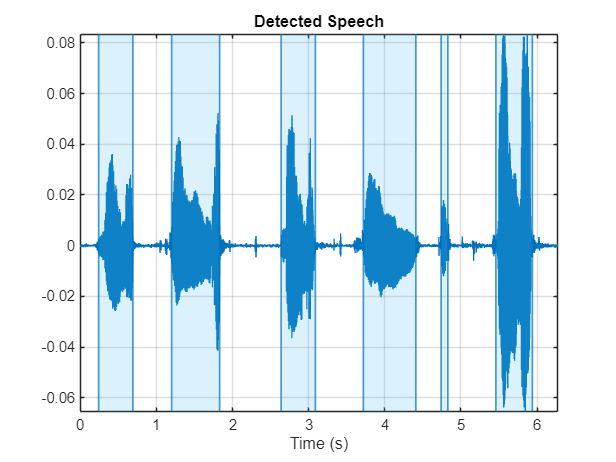Load two noise signals and `resample` to the audio sample rate.

```[noise200,fileFs200] = audioread("WashingMachine-16-8-mono-200secs.mp3"); [noise1000,fileFs1000] = audioread("WashingMachine-16-8-mono-1000secs.mp3"); noise200 = resample(noise200,fs,fileFs200); noise1000 = resample(noise1000,fs,fileFs1000);```

Use the supporting function `mixSNR` to corrupt the clean speech signal with washing machine noise at a desired SNR level in dB. Listen to the corrupted audio.

```SNR =-10; noisySpeech = mixSNR(speech,noise200,SNR); sound(noisySpeech,fs)```

Call `detectSpeech` on the noisy speech signal. The function fails to detect the speech regions given the very low SNR. The remainder of the example walks through training and evaluating deep learning-based VAD networks that can perform well under low SNR.

`detectSpeech(noisySpeech,fs)`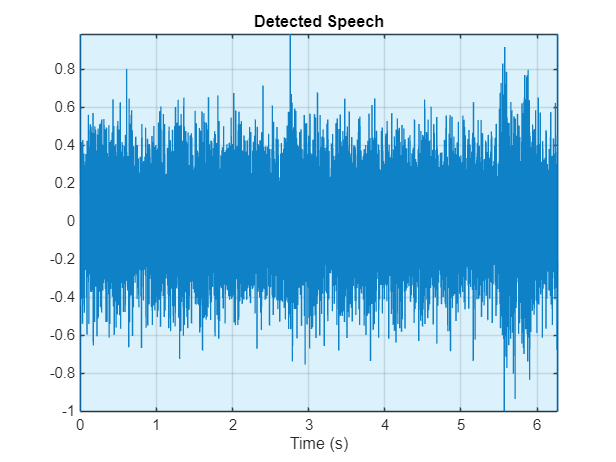```downloadFolder = matlab.internal.examples.downloadSupportFile("audio","google_speech.zip"); dataFolder = tempdir; unzip(downloadFolder,dataFolder) dataset = fullfile(dataFolder,"google_speech");```

Create `audioDatastore` objects to point to the training and validation data sets.

```adsTrain = audioDatastore(fullfile(dataset,"train"),IncludeSubfolders=true); adsValidation = audioDatastore(fullfile(dataset,"validation"),IncludeSubfolders=true);```

#### Construct Train and Validation Signals

The Google dataset consists of isolated words. Use the supporting function, `constructSignal`, to contruct train and validation signals that consist of isolated words and regions of silence. The `constructSignal` function also returns ground truth binary masks indicating the regions of speech in the train and validation signals.

```[audioTrain,TTrainPerSample] = constructSignal(adsTrain,fs,1000); [audioValidation,TValidationPerSample] = constructSignal(adsValidation,fs,200);```

Listen to the first 10 seconds of the constructed signal. Use `signalMask` and `plotsigroi` to visualize the signal and ground truth binary mask.

```duration =10; sound(audioTrain(1:duration*fs),fs) mask = signalMask(TTrainPerSample,SampleRate=fs); plotsigroi(mask,audioTrain,true) xlim([0,duration]) title("Clean Signal ("+duration+" seconds)")```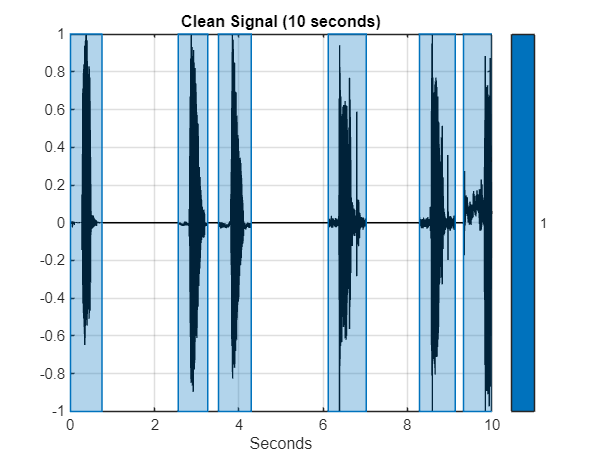#### Add Noise to Train and Validation Signals

Use the supporting function `mixSNR` to corrupt the train and validation signals with noise.

```audioTrain = mixSNR(audioTrain,noise1000,SNR); audioValidation = mixSNR(audioValidation,noise200,SNR);```

Listen to the first 10 seconds of the train signal and visualize the signal and mask.

```sound(audioTrain(1:duration*fs),fs) plotsigroi(mask,audioTrain,true) xlim([0,duration]) title("Training Signal ("+duration+" seconds)")```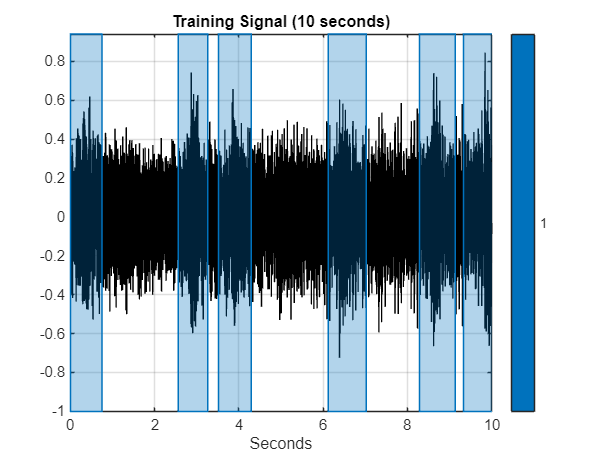### Input Pipeline

Define an `audioFeatureExtractor` to extract the following spectral features: `spectralCentroid`, `spectralCrest`, `spectralEntropy`, `spectralFlux`, `spectralKurtosis`, `spectralRolloffPoint`, `spectralSkewness`, `spectralSlope`, and the periodicity feature `harmonicRatio`. Extract features using a 256-point Hann window with 50% overlap.

```afe = audioFeatureExtractor(SampleRate=fs, ... Window=hann(256,"Periodic"), ... OverlapLength=128, ... ... spectralCentroid=true, ... spectralCrest=true, ... spectralEntropy=true, ... spectralFlux=true, ... spectralKurtosis=true, ... spectralRolloffPoint=true, ... spectralSkewness=true, ... spectralSlope=true, ... harmonicRatio=true); featuresTrain = extract(afe,audioTrain);```

Display the dimensions of the features matrix. The first dimension corresponds to the number of windows the signal was broken into (it depends on the signal length, window length, and overlap length). The second dimension is the number of features used in this example.

`[numWindows,numFeatures] = size(featuresTrain)`
```numWindows = 124999 ```
```numFeatures = 9 ```

In classification applications, it is a good practice to normalize all features to have zero mean and unity standard deviation.

Compute the mean and standard deviation for each coefficient, and use them to normalize the data.

```M = mean(featuresTrain,1); S = std(featuresTrain,[],1); featuresTrain = (featuresTrain - M) ./ S;```

Extract features from the validation signal using the same process.

```XValidation = extract(afe,audioValidation); XValidation = (XValidation - mean(XValidation,1)) ./ std(XValidation,[],1);```

Each feature corresponds to 256 samples of data (the window length), sampled every 128 samples (the hop length). For each window, set the expected voice/no voice value to the mode of the baseline mask values corresponding to those 256 samples. Convert the voice/no voice mask to categorical.

```windowLength = numel(afe.Window); overlapLength = afe.OverlapLength; TTrain = mode(buffer(TTrainPerSample,windowLength,overlapLength,"nodelay"),1); TTrain = categorical(TTrain);```

Do the same for the validation mask.

```TValidation = mode(buffer(TValidationPerSample,windowLength,overlapLength,"nodelay"),1); TValidation = categorical(TValidation);```

Use the supporting function `featureBuffer` to split the training features and the mask into sequences with a duration approximately 8 seconds and a 75% overlap between consecutive sequences.

```sequenceDuration =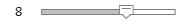8; analysisHopLength = numel(afe.Window) - afe.OverlapLength; sequenceLength = round(sequenceDuration*fs/analysisHopLength); overlapPercent =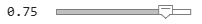0.75; XTrain = featureBuffer(featuresTrain',sequenceLength,overlapPercent); TTrain = featureBuffer(TTrain,sequenceLength,overlapPercent);```

### Network Architecture

LSTM networks can learn long-term dependencies between time steps of sequence data. This example uses the bidirectional LSTM layer `bilstmLayer` (Deep Learning Toolbox) to look at the sequence in both forward and backward directions.

```layers = [ ... sequenceInputLayer(afe.FeatureVectorLength) bilstmLayer(200,OutputMode="sequence") bilstmLayer(200,OutputMode="sequence") fullyConnectedLayer(2) softmaxLayer classificationLayer ];```

### Training Options

To define parameters for training, use `trainingOptions` (Deep Learning Toolbox). Use the Adam optimizer with a mini-batch size of 64 and a piecewise learn rate schedule.

```maxEpochs =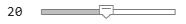20; miniBatchSize =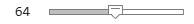64; options = trainingOptions("adam", ... MaxEpochs=maxEpochs, ... MiniBatchSize=miniBatchSize, ... Shuffle="every-epoch", ... Verbose=false, ... SequenceLength=sequenceLength, ... ValidationFrequency=floor(numel(XTrain)/miniBatchSize), ... ValidationData={XValidation.',TValidation}, ... Plots="training-progress", ... LearnRateSchedule="piecewise", ... LearnRateDropFactor=0.1, ... LearnRateDropPeriod=5, ... OutputNetwork="best-validation-loss");```

### Train Network

To train the network, use `trainNetwork` (Deep Learning Toolbox).

`speechDetectNet = trainNetwork(XTrain,TTrain,layers,options);`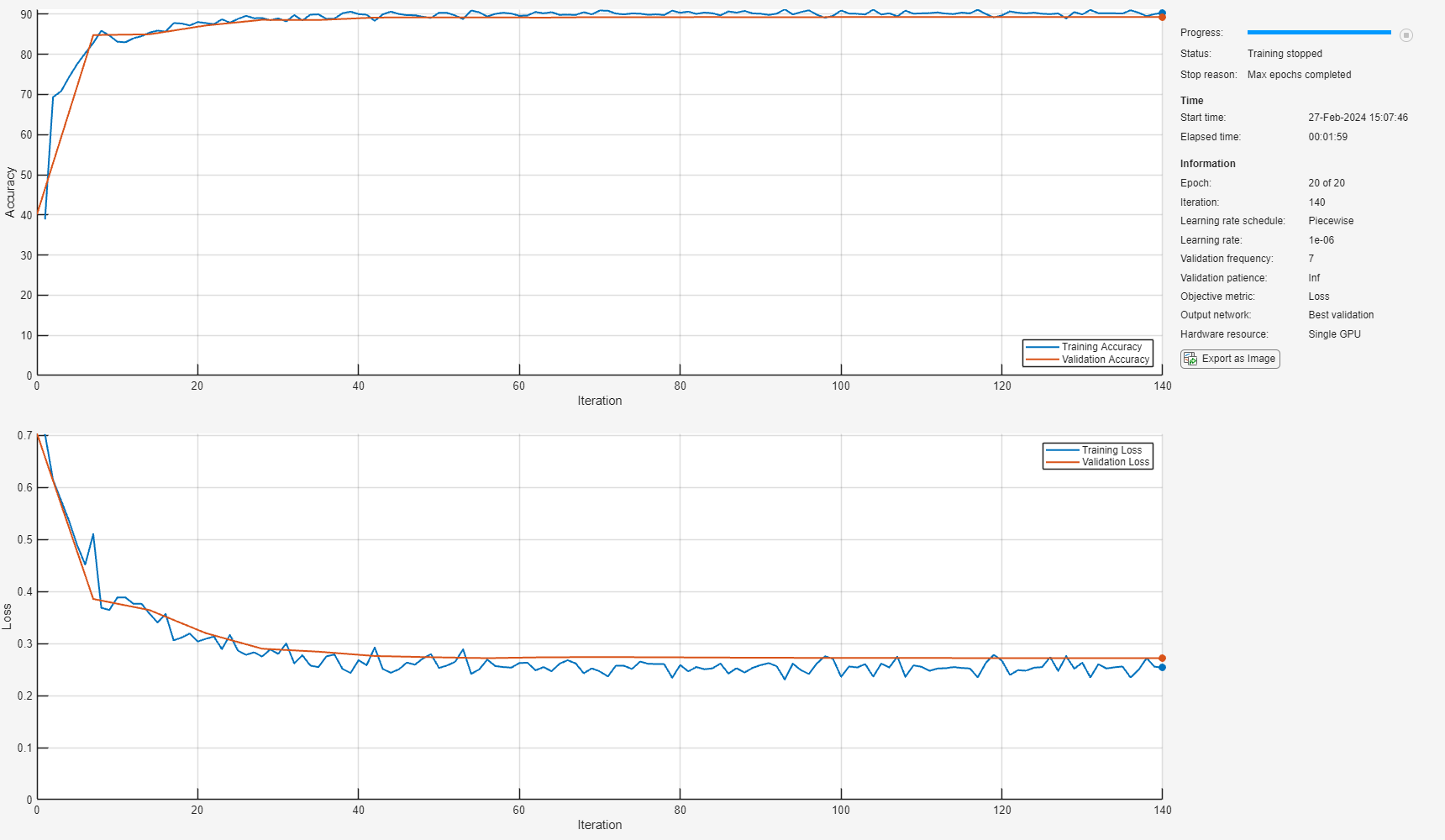### Evaluate Trained Network

Estimate voice activity in the validation signal using the trained network. Convert the estimated VAD mask from categorical to double, then replicate the window-based decisions to sample-based decisions.

```YValidation = classify(speechDetectNet,XValidation.'); YValidation = double(YValidation).' - 1; wL = numel(afe.Window); hL = wL - afe.OverlapLength; YValidationPerSample = [repelem(YValidation(1),floor(wL/2 + hL/2),1); repelem(YValidation(2:end-1),hL,1); repelem(YValidation(end),ceil(wL/2 + hL/2),1)];```

Calculate and plot the validation confusion matrix from the vectors of actual and estimated labels. Save the results for later analysis.

```cc = confusionchart(TValidationPerSample,YValidationPerSample, ... title="speechDetect - Validation Confusion Chart", ... ColumnSummary="column-normalized",RowSummary="row-normalized");```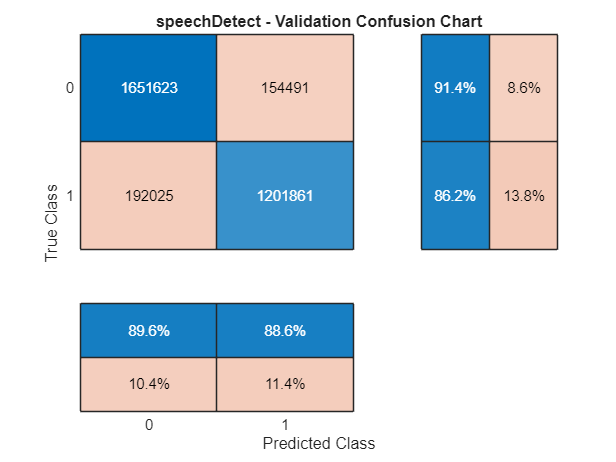`speechDetectResults = cc.NormalizedValues;`

The `vadnet` network is a pretrained network for voice activity detection. You can use it with the `vadnetPreprocess` and `vadnetPostprocess` functions for applications such as transfer learning, or you can use `detectspeechnn`, which encapsulates `vadnetPreprocess`, `vadnet`, and `vadnetPostprocess` for inference-only applications. The `vadnet` network performs well under every-day adverse conditions, however it fails in the cases of extreme SNR, such as the -10 dB SNR used in this example. Also, `vadnet` was trained to detect regions of continuous speech (meaning several words in a row), not isolated words. In short, the pretrained `vadnet` fails for the validation signal in this example.

`net = vadnet();`

Extract features from the validation signal using the same input pipeline used to train the network.

`XValidation = vadnetPreprocess(audioValidation,fs);`

`y = predict(net,XValidation);`

`vadnet` is a regression network and requires additional post-processing to determine decision boundaries. Use `vadnetPostprocess` to determine the boundaries of voice activity regions.

`boundaries = vadnetPostprocess(audioValidation,16e3,y);`

The `vadnetPostprocess` function returns the decisions as time boundaries. To convert the boundaries to a binary mask that corresponds to the original signal samples, use `sigroi2binmask`.

`YValidationPerSample = double(sigroi2binmask(boundaries,size(audioValidation,1)));`

To create a confusion chart to analyze the error, use `confusionchart` (Deep Learning Toolbox).

```confusionchart(TValidationPerSample,YValidationPerSample, ... title="vadnet - Validation Confusion Chart", ... ColumnSummary="column-normalized",RowSummary="row-normalized");```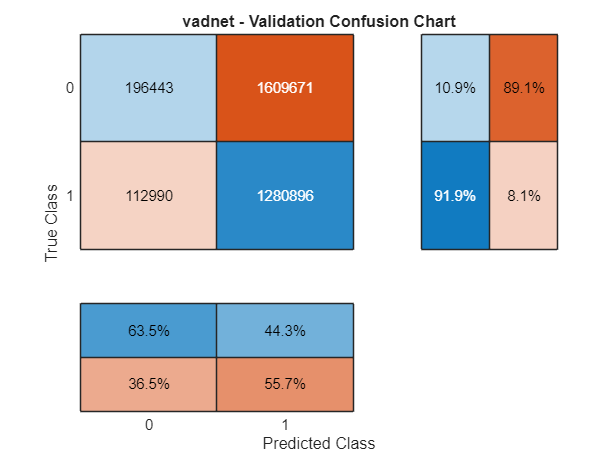### Transfer Learning

Apply transfer learning to the pretrained `vadnet` to make use of both the pretrained weights and the network architecture.

To extract the layer graph from the pretrained network, use `layerGraph` (Deep Learning Toolbox).

`layers = layerGraph(net);`

Extract features from the audio.

`featuresTrain = vadnetPreprocess(audioTrain,fs);`

Buffer the ground truth mask so that decisions correspond to the analysis windows used in `vadnetPreprocess`.

```windowLength = 400; overlapLength = 240; TTrainPerSamplePadded = [zeros(floor(windowLength/2),1);TTrainPerSample;zeros(ceil(windowLength/2),1)]; TTrain = mode(buffer(TTrainPerSamplePadded,windowLength,overlapLength,"nodelay"),1);```

```TValidationPerSamplePadded = [zeros(floor(windowLength/2),1);TValidationPerSample;zeros(ceil(windowLength/2),1)]; TValidation = mode(buffer(TValidationPerSamplePadded,windowLength,overlapLength,"nodelay"),1);```

Split the long training signal into overlapped sequences for training. Do the same for the ground-truth mask.

```sequenceDuration =8; analysisHopLength = windowLength - overlapLength; sequenceLength = round(sequenceDuration*fs/analysisHopLength); overlapPercent =0.75; XTrain = featureBuffer(featuresTrain,sequenceLength,overlapPercent); TTrain = featureBuffer(TTrain,sequenceLength,overlapPercent);```

To define parameters for training, use `trainingOptions` (Deep Learning Toolbox).

```miniBatchSize =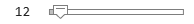12; maxEpochs =9; options = trainingOptions("adam", ... InitialLearnRate=0.01, ... LearnRateSchedule="piecewise", ... LearnRateDropPeriod=3, ... MiniBatchSize=miniBatchSize, ... Shuffle="every-epoch", ... ValidationFrequency=floor(numel(XTrain)/miniBatchSize), ... ValidationData={XValidation,TValidation}, ... Verbose=false, ... Plots="training-progress", ... MaxEpochs=maxEpochs, ... OutputNetwork="best-validation-loss" ... );```

To train the network, use `trainNetwork` (Deep Learning Toolbox).

`noisyvadnet = trainNetwork(XTrain,TTrain,layers,options);`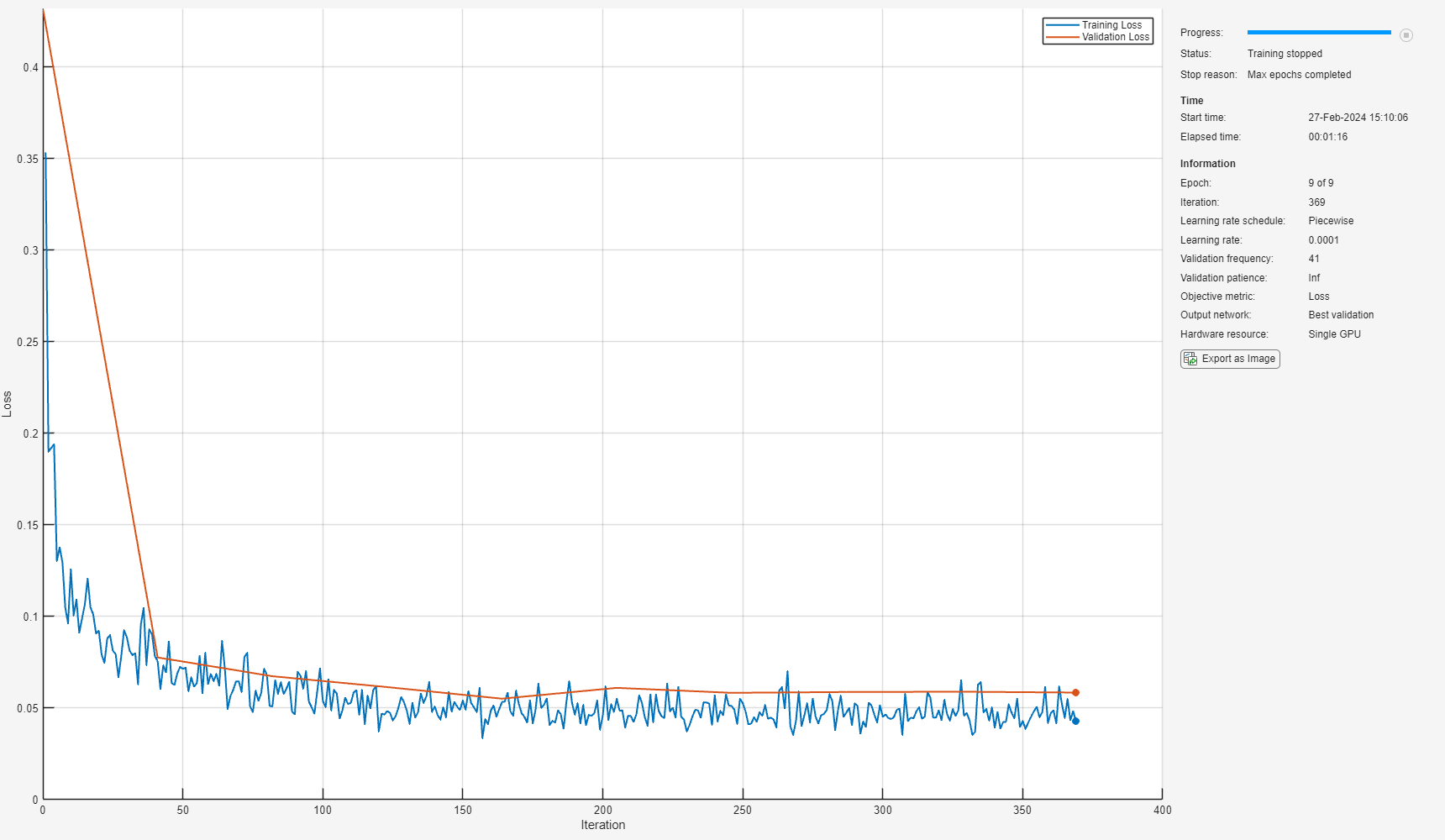Estimate voice activity in the validation signal using the trained network. Postprocess the predictions using `vadnetPostprocess`, then convert the boundaries in time to a sample-based mask.

```y = predict(noisyvadnet,XValidation); boundaries = vadnetPostprocess(audioValidation,fs,y); YValidationPerSample = double(sigroi2binmask(boundaries,size(audioValidation,1)));```

Calculate and plot the validation confusion matrix from the vectors of actual and estimated labels. Save the results for later analysis.

```cc = confusionchart(TValidationPerSample,YValidationPerSample, ... title="noisyvadnet - Validation Confusion Chart", ... ColumnSummary="column-normalized",RowSummary="row-normalized");```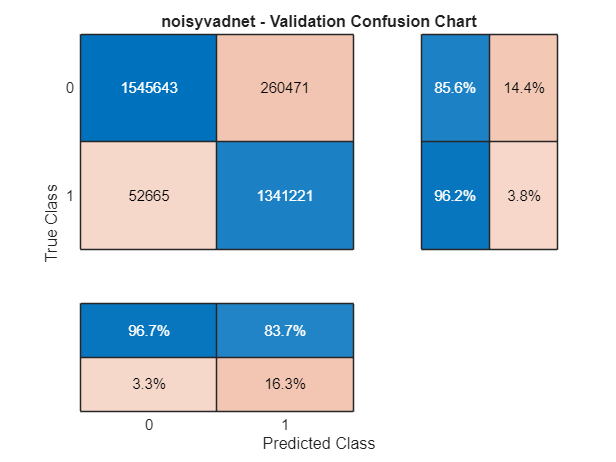`noisyvadnetResults = cc.NormalizedValues;`

### Compare Networks

There are several considerations when choosing a network, such as size, inference speed, error, and streaming capabilities.

#### Streaming

The `speechDetectNet` trained from scratch in this example is well-suited for streaming inference because its BiLSTM layers retain state between calls. See Voice Activity Detection in Noise Using Deep Learning for an example of using speechDetect for streaming voice activity detection.

The `vadnet` architecture consists of convolutional, recurrent, and fully-connected layers, and is not well-suited for low-latency streaming. See the `vadnet` documentation for an example of streaming VAD detection using `vadnet`.

#### Network Size

Compare the network sizes.

```networks = ["speechDetect","noisyvadnet"]; b = bar(reordercats(categorical(networks),networks),[whos("speechDetectNet").bytes/1024,whos("noisyvadnet").bytes/1024]); title("Network Size") ylabel("Size (KB)") grid on b.FaceColor = "flat"; b.CData(2,:) = [0.8500 0.3250 0.0980];```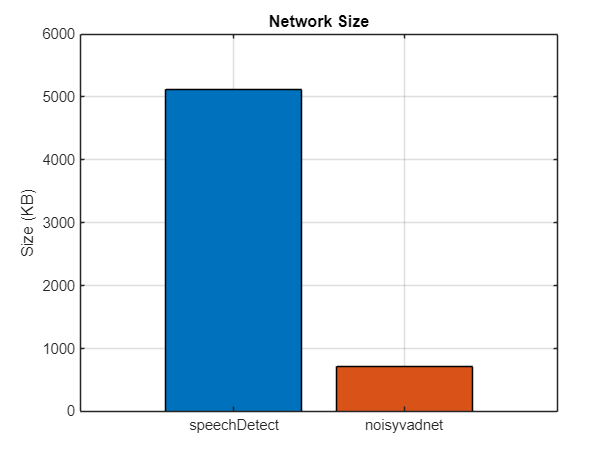#### Network Inference Speed

Compare the network inference speeds. The simple `speechDetect` architecture has faster inference speed on both the CPU and the GPU for short durations (approximately 8 second chunks or less). For longer durations, `speechDetect` is faster than `noisyvadnet` on the GPU and slower on the CPU.

```durationsToTest = [1,5,10,20,40]; environment = ["CPU","GPU"]; speechDetectSpeed = zeros(numel(durationsToTest),numel(environment)); noisyvadnetSpeed = zeros(numel(durationsToTest),numel(environment)); for jj = 1:numel(environment) for ii = 1:numel(durationsToTest) idx = 1:durationsToTest(ii)*fs; speechDetectFeatures = extract(afe,audioValidation(idx))'; vadnetFeatures = vadnetPreprocess(audioValidation(idx),fs); switch environment(jj) case "CPU" speechDetectSpeed(ii,1) = timeit(@()classify(speechDetectNet,speechDetectFeatures,ExecutionEnvironment="cpu"),1); noisyvadnetSpeed(ii,1) = timeit(@()predict(noisyvadnet,vadnetFeatures,ExecutionEnvironment="cpu"),1); case "GPU" speechDetectSpeed(ii,2) = gputimeit(@()classify(speechDetectNet,speechDetectFeatures,ExecutionEnvironment="gpu"),1); noisyvadnetSpeed(ii,2) = gputimeit(@()predict(noisyvadnet,vadnetFeatures,ExecutionEnvironment="gpu"),1); end end end tiledlayout(2,1) for ii = 1:numel(environment) nexttile plot(durationsToTest,speechDetectSpeed(:,ii),"b-", ... durationsToTest,noisyvadnetSpeed(:,ii),"r-", ... durationsToTest,speechDetectSpeed(:,ii),"bo", ... durationsToTest,noisyvadnetSpeed(:,ii),"ro") legend(["speechDetect","noisyvadnet"],Location="best") grid on xlabel("Audio Duration (s)") ylabel("Computation Duration (s)") title("Inference Speed ("+environment(ii)+")") end```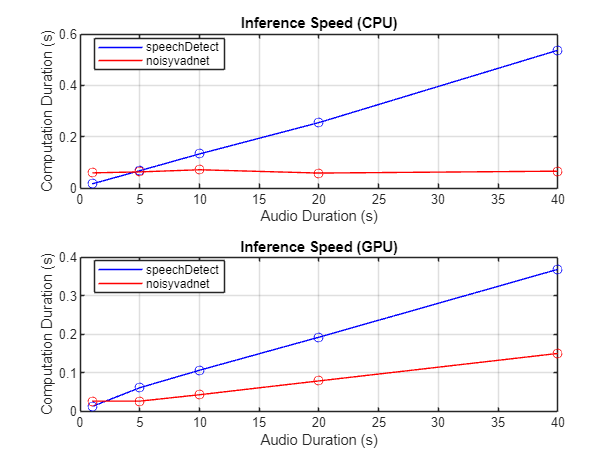#### Network Error

Use the previously calculated confusion charts to display common statistics for error analysis. Accuracy, recall, precision, and f1 score are all derived from the confusion matrices previously plotted.

Accuracy is defined as the ratio of correctly predicted observations to the total observations. It is the most intuitive metric but can be misleading for imbalanced data sets. For example, if speech is only present in 5% of the audio, then classifying all audio as non-speech would result in 95 % accuracy.

`$\mathrm{Accuracy}=\frac{\mathrm{TP}+\mathrm{TN}}{\mathrm{TP}+\mathrm{TN}+\mathrm{FP}+\mathrm{FN}}$`

Recall, also called sensitivity, is the ratio of correctly predicted positive observations to all observations that belong to the positive class. Recall answers the question: Of all speech regions, how many were correctly classified? A low recall indicates that regions of speech were misclassified as regions of nonspeech.

`$\mathrm{Recall}=\frac{\mathrm{TP}}{\mathrm{TP}+\mathrm{FN}}$`

Precision is the ratio of correctly predicted positive observations to the total predicted positive observations. Precision answers the question: Of all the observations the network classified as speech, how many were actually speech? A low precision indicates that regions of nonspeech were misclassified as regions of speech.

`$\mathrm{Precision}=\frac{\mathrm{TP}}{\mathrm{TP}+\mathrm{FP}}$`

F1 score is the harmonic mean of the precision and recall: it accounts for both false positives and false negatives.

`$\mathrm{F1}\text{\hspace{0.17em}}\mathrm{Score}=2\left(\frac{\mathrm{Precision}×\mathrm{Recall}}{\mathrm{Precision}+\mathrm{Recall}}\right)$`

The true measure of a network depends on your application. In real-world situations, a cost function is usually optimized which weights the costs of false positives and false negatives.

```TP = speechDetectResults(2,2); TN = speechDetectResults(1,1); FP = speechDetectResults(1,2); FN = speechDetectResults(2,1); speechDetectAccuracy = (TP+TN)/(TP+TN+FP+FN); speechDetectRecall = TP/(TP+FN); speechDetectPrecision = TP/(TP+FP); speechDetectF1Score = 2*(speechDetectRecall*speechDetectPrecision)/(speechDetectRecall+speechDetectPrecision); TP = noisyvadnetResults(2,2); TN = noisyvadnetResults(1,1); FP = noisyvadnetResults(1,2); FN = noisyvadnetResults(2,1); noisyvadnetAccuracy = (TP+TN)/(TP+TN+FP+FN); noisyvadnetRecall = TP/(TP+FN); noisyvadnetPrecision = TP/(TP+FP); noisyvadnetF1Score = 2*(noisyvadnetRecall*noisyvadnetPrecision)/(noisyvadnetRecall+noisyvadnetPrecision); figure bar(categorical(["Accuracy","Recall","Precision","F1 Score"]), ... [speechDetectAccuracy,noisyvadnetAccuracy; ... speechDetectRecall,noisyvadnetRecall; ... speechDetectPrecision,noisyvadnetPrecision; ... speechDetectF1Score,noisyvadnetF1Score]); title("Error Analysis") legend("speechDetect","noisyvadnet",Location="bestoutside") ylim([0.5,1]) grid on```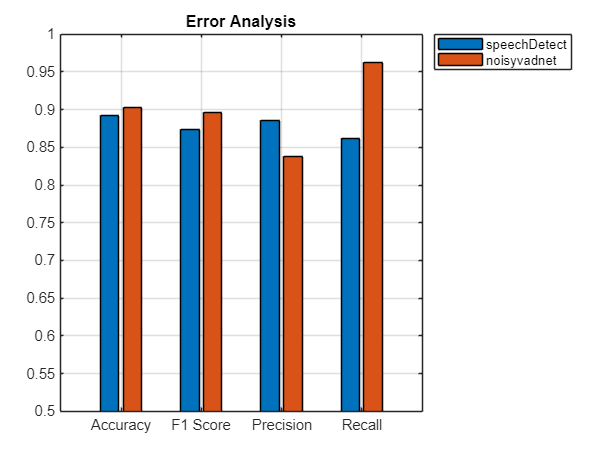### Supporting Functions

#### Convert Feature Vectors to Sequences

```function sequences = featureBuffer(features,featureVectorsPerSequence,overlapPercent) % y = featureBuffer(x,sequenceLength,overlapPercent) buffers a sequence of % feature vectors, x, into sequences of length sequenceLength overlapped by % overlapPercent. The sequences output are returns in a cell array for % consumption by trainNetwork. featureVectorOverlap = round(overlapPercent*featureVectorsPerSequence); hopLength = featureVectorsPerSequence - featureVectorOverlap; N = floor((size(features,2) - featureVectorsPerSequence)/hopLength) + 1; sequences = cell(N,1); idx = 1; for jj = 1:N sequences{jj} = features(:,idx:idx + featureVectorsPerSequence - 1); idx = idx + hopLength; end end```

#### Mix SNR

```function [noisySignal,requestedNoise] = mixSNR(signal,noise,ratio) % [noisySignal,requestedNoise] = mixSNR(signal,noise,ratio) returns a noisy % version of the signal, noisySignal. The noisy signal has been mixed with % noise at the specified ratio in dB. numSamples = size(signal,1); % Convert noise to mono noise = mean(noise,2); % Trim or expand noise to match signal size if size(noise,1)>=numSamples % Choose a random starting index such that you still have numSamples % after indexing the noise. start = randi(size(noise,1) - numSamples + 1); noise = noise(start:start+numSamples-1); else numReps = ceil(numSamples/size(noise,1)); temp = repmat(noise,numReps,1); start = randi(size(temp,1) - numSamples - 1); noise = temp(start:start+numSamples-1); end signalNorm = norm(signal); noiseNorm = norm(noise); goalNoiseNorm = signalNorm/(10^(ratio/20)); factor = goalNoiseNorm/noiseNorm; requestedNoise = noise.*factor; noisySignal = signal + requestedNoise; noisySignal = noisySignal./max(abs(noisySignal)); end```

#### Construct Signal

```function [audio,mask] = constructSignal(ds,fs,duration) % [audio,mask] = constructSignal(ds,fs,duration) constructs an audio signal % of the specified duration by concatenating samples from the % audioDatastore ds with random duration of silence between. win = hamming(50e-3*fs,"periodic"); % Create a 1000-second training signal by combining multiple speech files % from the training data set. Use detectSpeech to remove unwanted portions % of each file. Insert a random period of silence between speech segments. % Preallocate the training signal. N = duration*fs; audio = zeros(N,1); % Preallocate the voice activity training mask. Values of 1 in the mask % correspond to samples located in areas with voice activity. Values of 0 % correspond to areas with no voice activity. mask = zeros(N,1); % Specify a maximum silence segment duration of 2 seconds. maxSilenceSegment = 2; % Construct the training signal by calling read on the datastore in a loop. numSamples = 1; while numSamples < N data = read(ds); data = data ./ max(abs(data)); % Scale amplitude % Determine regions of speech idx = detectSpeech(data,fs,Window=win); % If a region of speech is detected if ~isempty(idx) % Extend the indices by five frames idx(1,1) = max(1,idx(1,1) - 5*numel(win)); idx(1,2) = min(length(data),idx(1,2) + 5*numel(win)); % Isolate the speech data = data(idx(1,1):idx(1,2)); % Write speech segment to training signal audio(numSamples:numSamples+numel(data)-1) = data; % Set VAD baseline mask(numSamples:numSamples+numel(data)-1) = true; % Random silence period numSilenceSamples = randi(maxSilenceSegment*fs,1,1); numSamples = numSamples + numel(data) + numSilenceSamples; end end audio = audio(1:N); mask = mask(1:N); end```